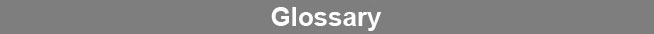Select Page### Fit Probability

The Fit probability (Fit Prob) is a statistical measure of how well a weighted curve regression fits a set of data. The Fit probability is the chi squared probability of the residual sum of squared errors (RSSE) at number of points minus number of coefficients degrees of freedom.www.brendan.com